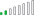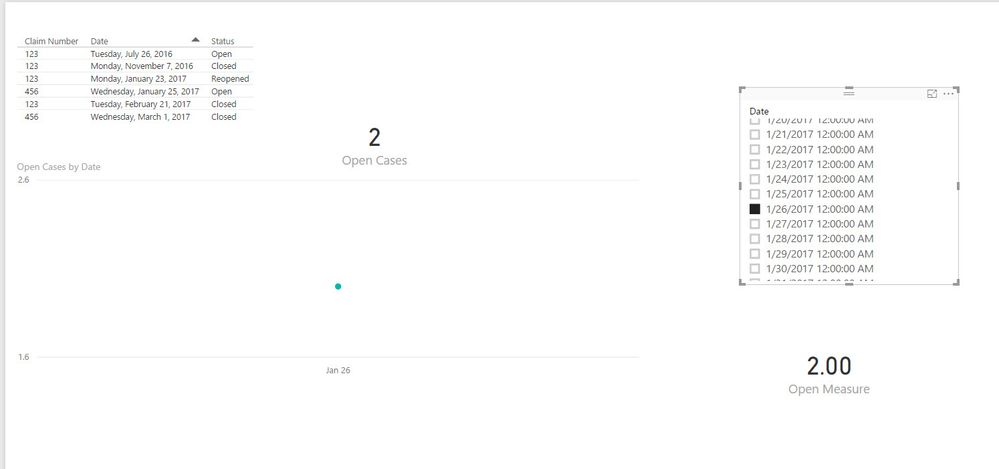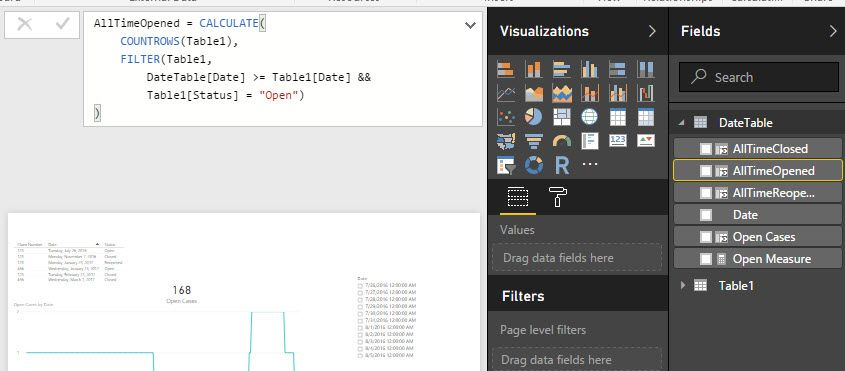cancel
Showing results for
Did you mean:Frequent Visitor

## Dynamic Column Count Based on Date Slicer Selection

I have a claims history table with multiple rows for each claim number.  Each row provides the claim number, the status of the claim, and a date when that status occurred.  I'm trying to count the number of claims that are currently open/reopened based on a date slicer the user can control.  A simple view of my data:

 Claim Number Status Date Rank 123 Open 7/26/2016 1 123 Close 11/7/2016 2 123 Reopened 1/23/2017 3 123 Closed 2/21/2017 4 456 Open 1/25/2017 1 456 Closed 3/1/2017 2

I added a rank column thinking it might help me select the max rank as of a given date, which could then be linked to the status but I haven't been able to figure out how to do that.

What I am trying to get at :

given the data in the above table if a user selected via a slicer (DimDate[Date] serves as the field for the slicer) the following dates, I would return the following number of claims.

 On Date # of Open Claims 8/1/2016 1 12/1/2016 0 1/25/2017 2 2/27/2017 1

Any help on creating the measure for this would be greatly appreciated.

1 ACCEPTED SOLUTIONResolver II

Here's my thought on how to tackle this.  I created your table in Excel and imported it into PBI, then did the following:

• Created a date table
`DateTable = Calendar(Minx(table1,Table1[Date]),Now()) `

• To the date table I added 3 calculated columns, purpose being to count how many claims have been opened, reopened, and closed all time as of that date:
• ```AllTimeOpened = CALCULATE(
COUNTROWS(Table1),
FILTER(Table1,
DateTable[Date] >= Table1[Date] &&
Table1[Status] = "Open")
)```
```AllTimeReopened = CALCULATE(
COUNTROWS(Table1),
FILTER(Table1,
DateTable[Date] >= Table1[Date] &&
Table1[Status] = "Reopened")
)```
```AllTimeClosed = CALCULATE(
COUNTROWS(Table1),
FILTER(Table1,
DateTable[Date] >= Table1[Date] &&
Table1[Status] = "Closed")
)```
• Then to the date table, I added another calculated column called "Open Cases"
• ```Open Cases = CALCULATE(
VALUES(DateTable[AllTimeOpened]) +
VALUES(DateTable[AllTimeReopened]) -
VALUES(DateTable[AllTimeClosed] )
)```

The result of all this is that I have a count in my date table of how many actively open cases there are on any given day.  I can chart this out, but if I try to make a card/slicer it gives me inaccurate data because it sums up my column.  Instead I probably want to make it a calculated measure instead.  The measure will only be meaningful if a date slicer is used, so I wrote it as such:

```Open Measure = IFERROR(
CALCULATE(
VALUES(DateTable[AllTimeOpened]) +
VALUES(DateTable[AllTimeReopened]) -
VALUES(DateTable[AllTimeClosed] )
),
"Use Slicer")```

Might be a better way to do that.

Anyway, here are the visual results:Is this on the right path of what you're looking for?

Dan

9 REPLIES 9Resolver II

Here's my thought on how to tackle this.  I created your table in Excel and imported it into PBI, then did the following:

• Created a date table
`DateTable = Calendar(Minx(table1,Table1[Date]),Now()) `

• To the date table I added 3 calculated columns, purpose being to count how many claims have been opened, reopened, and closed all time as of that date:
• ```AllTimeOpened = CALCULATE(
COUNTROWS(Table1),
FILTER(Table1,
DateTable[Date] >= Table1[Date] &&
Table1[Status] = "Open")
)```
```AllTimeReopened = CALCULATE(
COUNTROWS(Table1),
FILTER(Table1,
DateTable[Date] >= Table1[Date] &&
Table1[Status] = "Reopened")
)```
```AllTimeClosed = CALCULATE(
COUNTROWS(Table1),
FILTER(Table1,
DateTable[Date] >= Table1[Date] &&
Table1[Status] = "Closed")
)```
• Then to the date table, I added another calculated column called "Open Cases"
• ```Open Cases = CALCULATE(
VALUES(DateTable[AllTimeOpened]) +
VALUES(DateTable[AllTimeReopened]) -
VALUES(DateTable[AllTimeClosed] )
)```

The result of all this is that I have a count in my date table of how many actively open cases there are on any given day.  I can chart this out, but if I try to make a card/slicer it gives me inaccurate data because it sums up my column.  Instead I probably want to make it a calculated measure instead.  The measure will only be meaningful if a date slicer is used, so I wrote it as such:

```Open Measure = IFERROR(
CALCULATE(
VALUES(DateTable[AllTimeOpened]) +
VALUES(DateTable[AllTimeReopened]) -
VALUES(DateTable[AllTimeClosed] )
),
"Use Slicer")```

Might be a better way to do that.

Anyway, here are the visual results:Is this on the right path of what you're looking for?

DanFrequent Visitor

@danrmcallister I'm running into an error with trying to create the measure - it's telling me that the syntax is incorrect.  Any ideas on why I might be getting that error?

My Measure in the Date Table:

Open Measure = CALCULATE(
VALUES(Date[AllTimeOpened])+
VALUES('Date'[AllTimeReopened]) -
VALUES('Date'[AllTimeClosed]))

ERROR:

The syntax for '[AllTimeOpened]' is incorrect. (DAX(CALCULATE( VALUES(Date[AllTimeOpened])+ VALUES('Date'[AllTimeReopened]) - VALUES('Date'[AllTimeClosed])))).Resolver II

The "AllTimeOpened", "AllTimeReopened", and "AllTimeClosed" fields you see below were calculated columns I created against my date table.  Did you complete that step first?Frequent Visitor

@danrmcallister I created all three of the calculated columns, and I just realized the VERY basic obvious reason why I was getting a syntax error.  I didn't have Date in quotes for the first VALUES in my measure.   I have now fixed that and my error is gone.  I can now officially test the code.  Thank you again for your time!Frequent Visitor

@danrmcallister The good news - I was able to get this to work, and the logic works for filtering by date.  The problem I'm realizing is that I can't have the counts in the Date table because if the user wants to filter the data by anything else (additional fields that I didn't show but are common for the user to bring into the Data table like cause of loss) they won't be able to filter by any additional fields.  Thank you for all of your time.Resolver II

Did you try creating a relationship between your date table and your fact table based on the date?  It should work!Frequent Visitor

@danrmcallister I think I must be missing something simple.  I have a relationship between my Date table and Fact table (link is on the Date).  I have two filters on my sheet, the first is a 'Date'[Date] filter.  The second is a FactTable[loss cause] filter.  My claims count changes if the Date filter changes (and it changes the options available on my loss cause filter).  However, nothing happens to the open claims count if I select a single specific loss cause.  With the sample you created, if you added a column in Table1 titled Loss Cause and give claim 123 a cause of "trip" and claim 456 a cause of "accident", are you able to add a second filter for Loss Cause and have it work?Frequent Visitor

@danrmcallister I got it to work the way I need based on your suggestions - thank you so much for your help!  I created three measures in my fact table that gave me a total of open, reopened, and closed claims (code provided for one of those measures).  Then I created a measure based on those three measures: adding total open to reopened and subtracts claims, which interacts with both a date and loss cause filter.

Count Closes =
VAR EndingDate =
MAX ( 'Date'[Date] )
RETURN
( CALCULATE (COUNTROWS (FactTable), 'Date'[Date] <= EndingDate, FactTable[Status] = "Closed"))Frequent Visitor

@danrmcallister Thank you for the response!  Appears this should get me what I need.  Putting it in now to test.  Will let you know final verdict.Announcements#### Exclusive opportunity for Women!

Join us for a free, hands-on Microsoft workshop led by women trainers for women where you will learn how to build a Dashboard in a Day!#### Power Platform Conference-Power BI and Fabric Sessions

Join us Oct 1 - 6 in Las Vegas for the Microsoft Power Platform Conference.Top Solution Authors
Top Kudoed Authors
Users online (4,097)